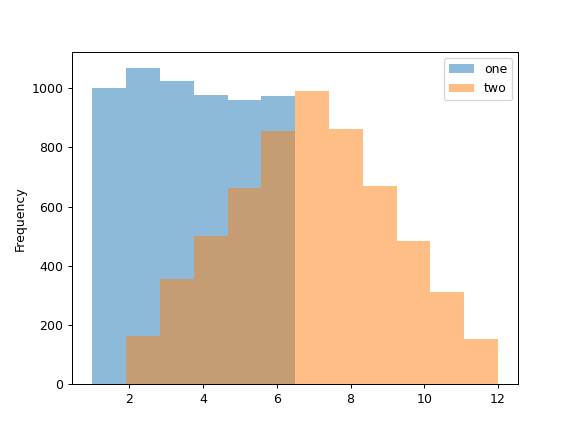# pandas.Series.plot.hist¶

Series.plot.hist(by=None, bins=10, **kwargs)[source]

Draw one histogram of the DataFrame’s columns.

A histogram is a representation of the distribution of data. This function groups the values of all given Series in the DataFrame into bins and draws all bins in one `matplotlib.axes.Axes`. This is useful when the DataFrame’s Series are in a similar scale.

Parameters
bystr or sequence, optional

Column in the DataFrame to group by.

binsint, default 10

Number of histogram bins to be used.

**kwargs

Additional keyword arguments are documented in `DataFrame.plot()`.

Returns
class:matplotlib.AxesSubplot

Return a histogram plot.

`DataFrame.hist`

Draw histograms per DataFrame’s Series.

`Series.hist`

Draw a histogram with Series’ data.

Examples

When we draw a dice 6000 times, we expect to get each value around 1000 times. But when we draw two dices and sum the result, the distribution is going to be quite different. A histogram illustrates those distributions.

```>>> df = pd.DataFrame(
...     np.random.randint(1, 7, 6000),
...     columns = ['one'])
>>> df['two'] = df['one'] + np.random.randint(1, 7, 6000)
>>> ax = df.plot.hist(bins=12, alpha=0.5)
```